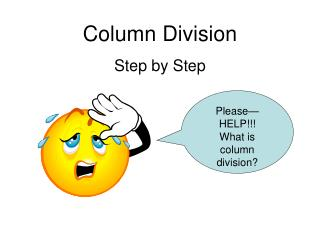DownloadDownload PresentationColumn Division Step by Step

# Column Division Step by Step

Download Presentation## Column Division Step by Step

- - - - - - - - - - - - - - - - - - - - - - - - - - - E N D - - - - - - - - - - - - - - - - - - - - - - - - - - -
##### Presentation Transcript

1. Column Division Step by Step Please—HELP!!! What is column division?

2. 479 / 9 = ? Set up your problem as usual but spread out the numbers in the dividend. 9 4 7 9

3. 479 / 9 = ? Draw lines between the numbers in the dividend. 9 4 7 9

4. 479 / 9 = ? How many groups of 9 are there in 4? 9 4 7 9

5. 479 / 9 = ? How many groups of 9 are there in 4? There are none because 9 is bigger than 4. Put a 0 above the 4 and a 0 below the 4. • 0 • 7 9 • 0 9

6. 479 / 9 = ? Subtract 0 from 4. 0 4 7 9 0 4 9

7. 479 / 9 = ? Move the 4 in front of the 7. 0 4 47 9 0 4 9

8. 479 / 9 = ? How many groups of 9 can you find in 47? 0 4 47 9 0 4 9

9. 479 / 9 = ? Since 9 * 5 is 45, I know there are 5 groups of 9 in 47. Put the 5 above the 7 and 45 below 47 and subtract. 0 5 4 47 9 0 4 5 4 2 9

10. 479 / 9 = ? Move the 2 in front of the 9. 0 5 4 4 7 29 0 4 5 4 2 9

11. 479 / 9 = ? How many groups of 9 can you find in 29? 0 5 4 4 7 29 0 4 5 4 2 9

12. 479 / 9 = ? Since 9 * 3 is 27, I know there are 3 groups of 9 in 29. Put the 3 above the 9 and 27 below 29 and subtract. 0 5 3 4 4 7 29 0 4 5 2 7 4 2 2 9

13. 479 / 9 = ? Since there is not another number to place the 2 in front of, it must be the remainder. My answer is 53 r 2. 0 5 3 4 4 7 2 9 0 4 5 2 7 4 2 2 9

14. 479 / 9 = 53 r 2